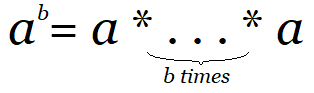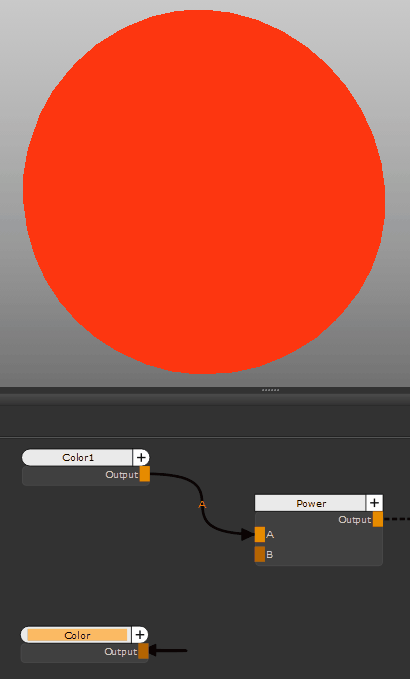# Power Node

Access: NodesMathPowerThe Power node outputs the value of A raised to the power B. This can be useful to raise base color values to become darker or lighter, depending on the exponent.

For the inputs A = [R G B A] and B = [X Y Z W], the Power node performs the following calculation:

RX, GY, BZ, AW.

# Power Node Examples

 Input (RGBA) Calculation Output (RGBA) Input A = 1.0, 0.213, 0.063, 1.0 Input B = 1.0, 0.454, 0.454, 1.0 R = 1.01.0 G = 0.2130.454 B = 0.0630.454 A = 1.01.0 1.0, 0.495, 0.285, 1.0 Input A = 0.5, 0.9, 0.1, 1.0 Input B = 0.1, 0.1, 0.1, 1.0 R = 0.50.1 G = 0.90.1 B = 0.10.1 A = 1.01.0 0.933, 0.989, 0.794, 1.0A Color node with a value of 1.0, 0.213, 0.063 raised to the power of a Color node with a value of 1.0, 0.454, 0.454.

# Power Node Inputs

 A The value to raise to the power B. When no node is connected, the value defaults to the value specified in Default Input A. B The power to raise A to. When no node is connected, the value defaults to the value specified in Default Input B.

# Power Node Properties

 Default Input A text field, slider The value to use for A if no node is connected to the A input. Default Input B text field, slider The value to use for B if no node is connected to the B input. R checkbox If checked, the red component of A is raised to the red component of power B. If unchecked, the red component is passed through to the output as the original value of input A. G checkbox If checked, the green component of A is raised to the green component of power B. If unchecked, the green component is passed through to the output as the original value of input A. B checkbox If checked, the blue component of A is raised to the blue component of power B. If unchecked, the blue component is passed through to the output as the original value of input A. A checkbox If checked, the alpha component of A is raised to the alpha component of power B. If unchecked, the alpha component is passed through to the output as the original value of input A.